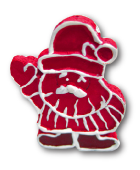### Challenge from 5. December

Number Game
Since there was some confusion about the formulation of this challenge:

The elves Alpha, Beta, Gamma each get exactly one card. On each of these cards, there is exactly one number. These three numbers are pairwise distinct numbers from 1, 2, 3, 4, 5, 6, 7, 8, 9, 10. The largest of the three numbers is the sum of the smaller two.

Authors: Aart Blokhuis, Gerhard Woeginger

Project: 4TU.AMI

Challenge:

Santa Clause tells the three super-smart elves Alpha, Beta, and Gamma, “Each of you has received a card that carries a number from 1, 2, 3, 4, 5, 6, 7, 8, 9, 10. Your three numbers are pairwise distinct, and the largest one equals the sum of the two smaller ones. Now, please have a look at your cards with your number, but don't show it to the two others!”

Alpha, Beta and Gamma stare at their cards for some time.

1. After some time Alpha says, “According to my knowledge, there are at most eight possible candidates for Beta's number.”

2. Then, Beta says, “According to my current knowledge, there are exactly three possible candidates for Gamma's number.”

3. Gamma shouts, “I see! Now, I know Alpha's number.”

4. Alpha thinks about it and says, “I still don't know Beta's number.”

5. Beta exclaims, “I see! Now, I also do know Alpha's number.”

Of course, we would like to know: What is Alpha's number?

Artwork: Frauke Jansen1. Alpha's number is 1.

2. Alpha's number is 2.

3. Alpha's number is 3.

4. Alpha's number is 4.

5. Alpha's number is 5.

6. Alpha's number is 6.

7. Alpha's number is 7.

8. Alpha's number is 8.

9. Alpha's number is 9.

10. Alpha's number is 10.